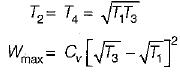Courses

# Test: Air Standard Cycles - 3

## 10 Questions MCQ Test Topicwise Question Bank for Mechanical Engineering | Test: Air Standard Cycles - 3

Description
This mock test of Test: Air Standard Cycles - 3 for Mechanical Engineering helps you for every Mechanical Engineering entrance exam. This contains 10 Multiple Choice Questions for Mechanical Engineering Test: Air Standard Cycles - 3 (mcq) to study with solutions a complete question bank. The solved questions answers in this Test: Air Standard Cycles - 3 quiz give you a good mix of easy questions and tough questions. Mechanical Engineering students definitely take this Test: Air Standard Cycles - 3 exercise for a better result in the exam. You can find other Test: Air Standard Cycles - 3 extra questions, long questions & short questions for Mechanical Engineering on EduRev as well by searching above.
QUESTION: 1

### For the same peak pressure and heat input

Solution: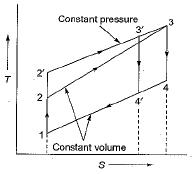Otto cycle ⇒ 1-2-3-4
Diesel cycle 1-2'-3'-4'
It is clear from T - s diagram that the heat rejected by the Diesel cycle (area below 4'-1) is less then the heat rejection by the Otto cycle (area below 4-1); hence the diesel cycle is more efficient than Otto cycle for the condition of same maximum pressure heat input. The Dual cycle efficiency lies between the Otto and Diesel cycle.

QUESTION: 2

### For the same peak pressure and work output

Solution: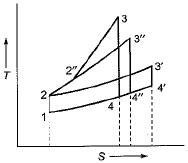The efficiency is given by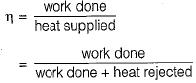The work done in Otto and Diesel cycles (areas 1 -2-3-4 and 1-2-3'-4' respectively) can be equal only if the point 3' has a greater entropy than 3 and in that Case the heat rejected by the diesel cycle is less than that of Otto cycle. Hence, for these conditions, diesel cycle is more efficient than Otto cycle and Dual cycle will be in between these two.

QUESTION: 3

### Time loss factor in actual cycle is due to

Solution:
QUESTION: 4

Air standard diesel cycle has a compression ratio of 14 and cut off takes place at 6% of the stroke. What is the cut off ratio?

Solution:

V3/V2 = Cut - off ratio
V1/ V2 = r = Compression ratio = 14
V1 = 14 V2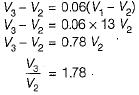QUESTION: 5

A single cylinder compression ignition engine, operating on the air-standard diesel cycle, has a mean effective pressure of 1.0 MPa and a compression ratio of 21. The engine has a clearance volume of 5 x 10-5m3. The heat added at constant pressure is 2.0 kJ. The thermal efficiency of the engine is

Solution: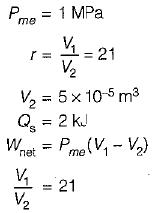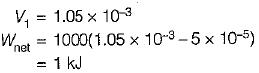Thermal efficiency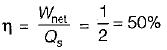QUESTION: 6

Match List-I with List-ll and select the correct answer using the codes given below the lists:
List - I
A. Compression ratio
B. Pressure ratio
C. Cut-off ratio
D. Expansion ratio
List - II
1. Brayton cycle
2. Diesel cycle
3. Dual combustion cycle
4. Otto cycle
Codes:
A B C D
(a) 1 4  3 2
(b) 1 4  2 3
(c) 4 1  2 3
(d) 4 1 3  2

Solution:
QUESTION: 7

In a spark ignition working on the ideal Otto cycle, the compression ratio is 5.5. The work output per cycle (i.e., area of the P - V diagram) is equal to 23.625 x 105 x VcJ, where Vc is the clearance volume in m3. The indicated mean effective pressure is

Solution:

Compression ratio
r = V/Vc = 5.5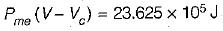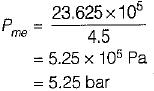QUESTION: 8

A turbocharged four-stroke direct injection diesel engine has a displacement volume of 0.0259 m3 (25.9 litres). The engine has an output of 950 kW at 2200 rpm. The mean effective pressure (in MPa) is closest to

Solution: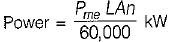For four stroke engine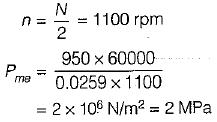QUESTION: 9

Which one of the following is NOT a necessary assumption for the air-standard Otto cycle?

Solution:

Air-standard cycle, which is based on the following assumptions:
(i) The working medium is assumed to be a perfect gas and follows the relation pV = mRT or p = ρRT.
(ii) There is no change in the mass of the working medium.
(iii) All the processes that constitute the cycle are reversible.
(iv) Heat is assumed to be supplied from a constant high temperature source and not from chemical reactions during the cycle.
(v) The working medium has constant specific heats throughout the cycle.

QUESTION: 10

For maximum specific output of a constant volume cycle (Otto cycle)

Solution:

Specific work - Cv(T3 - T4) - Cv(T2 - T1)